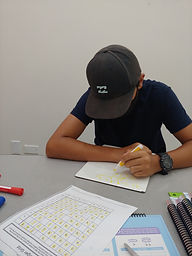## Ms. Mari

### Target 1​

Review

Number Operation

:

Computation

###### 1:

Apply the procedure for adding integers with the same signs.

###### 2:

Apply the procedure for adding integers with unlike signs.

###### 3:

Subtract numbers with different signs, changing the sign of the number and arithmetic operation to calculate.

6th

###### Vocabulary:

INTEGER

Activities:

Completed a review of adding and subtracting integers by filling in blanks on a puzzle, in which all the numbers in each row added up to the number at the end of the row, and all of the numbers in each column added up to the number at the bottom of the column.### Home Exploration

###### Guiding Questions:## Absent Students:

### Target 2

:

###### 1:

Understand that exponential functions are functions made of an exponential expression.

###### 2:

Analyze the relationships between numbers in a sequence to determine the exponential expression.

HS

###### Vocabulary:

EXPONENTIAL FUNCTION

Activities:

- Modeled a relationship using a piece of string.

(1) Folded the string in half and continuously cut the string through the middle, comparing number of cuts to number of pieces after each cut

(2) Continuously folded the string and made one cut, comparing number of folds to number of pieces after the cut

(3) Continuously folded the string and made the same number of cuts as folds, comparing the number of folds/cuts to the number of pieces after the cut

- Determined a rule for the relationship and whether the relationship was exponential.

- Graphed the results on a coordinate plane and observed the shape of the graph.### Home Exploration

###### Guiding Questions:### Target 3

:

###### Vocabulary:

Activities:### Home Exploration